# 机器学习聚类算法之HDBSCAN

HDBSCAN – Hierarchical Density-Based Spatial Clustering of Applications with Noise. Performs DBSCAN over varying epsilon values and integrates the result to find a clustering that gives the best stability over epsilon. This allows HDBSCAN to find clusters of varying densities (unlike DBSCAN), and be more robust to parameter selection.

• 由于需要在整个数据空间构建树，算法需要很大的IO开销
• 算法输入参数没有一个很完美的科学标准来作为参考，这就使得人为干扰的因素变得很大，参数选取略有偏差对于聚类的效果有时会呈现出完全不同的效果

HDBSCAN算法是对OPTICS算法的一种改进，但并不是没有缺点。比如其对于边界点的处理方面效果却不是很理想。

## HDBSCAN算法原理

import numpy as np
import matplotlib.pyplot as plt
import seaborn as sns
import sklearn.datasets as data
%matplotlib inline
sns.set_context('poster')
sns.set_style('white')
sns.set_color_codes()
plot_kwds = {'alpha' : 0.5, 's' : 80, 'linewidths':0}

moons, _ = data.make_moons(n_samples=50, noise=0.05)
blobs, _ = data.make_blobs(n_samples=50, centers=[(-0.75,2.25), (1.0, 2.0)], cluster_std=0.25)
test_data = np.vstack([moons, blobs])
plt.scatter(test_data.T, test_data.T, color='b', **plot_kwds)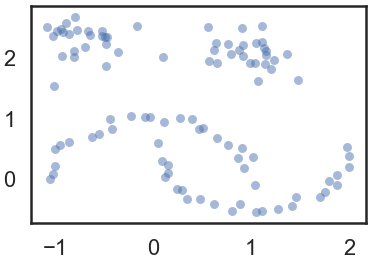HDBSCAN的使用方式

import hdbscan

clusterer = hdbscan.HDBSCAN(min_cluster_size=5, gen_min_span_tree=True)
clusterer.fit(test_data)


• 根据密度/稀疏度对空间进行变换
• 建立距离加权图的最小生成树
• 构造连接组件的簇层次结构
• 根据最小的簇大小压缩簇层次结构
• 从压缩树中提取稳定的簇

### 变换空间

$$core_k(x) = d(x,N^{\mathcal{k}}(x))$$

$$d_{\mathrm{mreach-}k}(a,b) = \max \{\mathrm{core}_k(a), \mathrm{core}_k(b), d(a,b) \}$$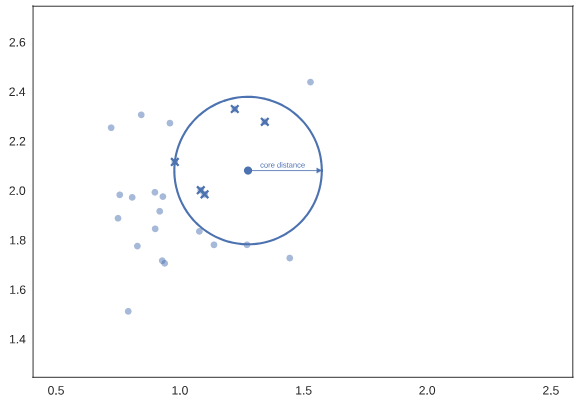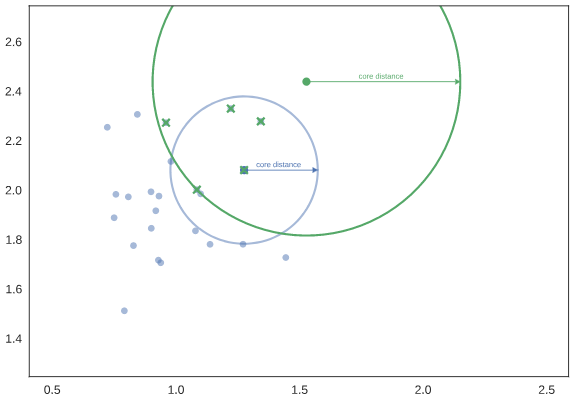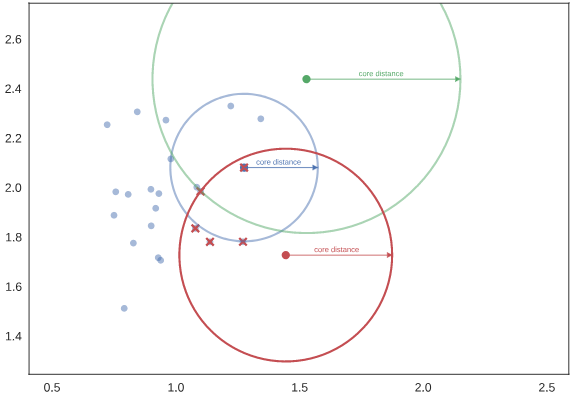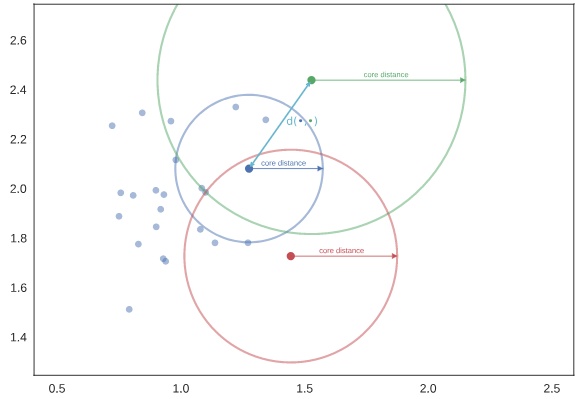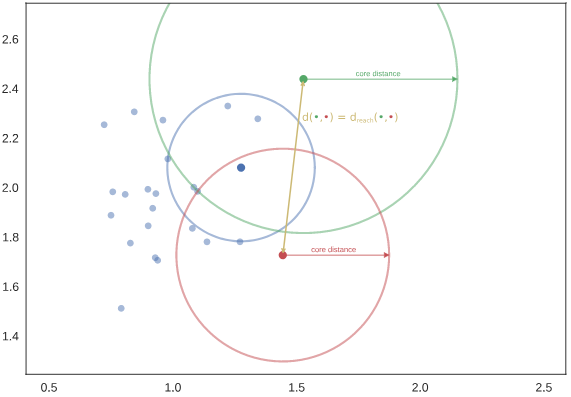### 建立最小生成树

clusterer.minimum_spanning_tree_.plot(edge_cmap='viridis',edge_alpha=0.6,node_size=80,edge_linewidth=2)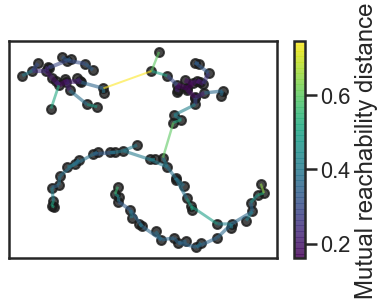### 构建簇层次结构

clusterer.single_linkage_tree_.plot(cmap='viridis', colorbar=True)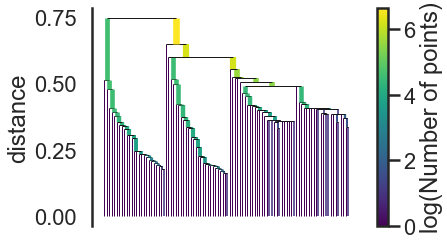clusterer.condensed_tree_.plot()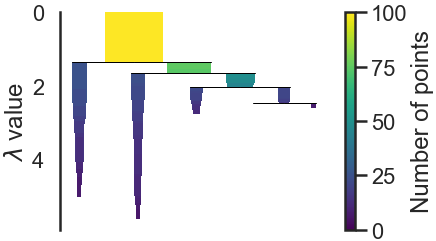### 提取簇

$$s_{\mathrm{cluster}} = \sum_{p \in \mathrm{cluster}} (\lambda_p – \lambda_{\mathrm{birth}})$$

• $\lambda_{\mathrm{birth}}$：团簇形成时的$\lambda$值
• $\lambda_{\mathrm{death}}$：团簇分裂为两个子团簇时的$\lambda$值
• $\lambda_p$：从团簇中分离出去时的$\lambda$值

clusterer.condensed_tree_.plot(select_clusters=True, selection_palette=sns.color_palette())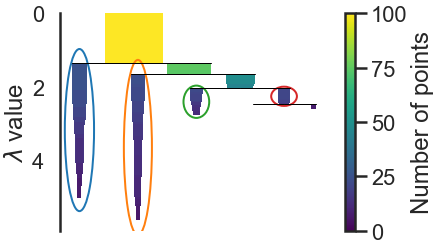palette = sns.color_palette()
cluster_colors = [sns.desaturate(palette[col], sat)
if col >= 0 else (0.5, 0.5, 0.5) for col, sat in
zip(clusterer.labels_, clusterer.probabilities_)]
plt.scatter(test_data.T, test_data.T, c=cluster_colors, **plot_kwds)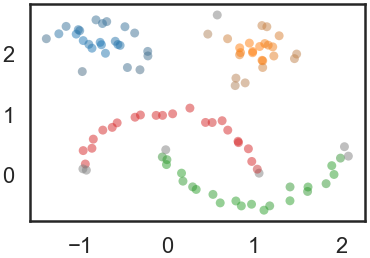## HDBSCAN使用实例

import numpy as np
import pandas as pd
import hdbscan
import matplotlib.pyplot as plt
import matplotlib.cm as cm
from math import pi, cos, sin, atan2, sqrt

def get_centroid(cluster):
x = y = z = 0
coord_num = len(cluster)
for coord in cluster:
lat = coord * pi / 180
lon = coord * pi / 180

a = cos(lat) * cos(lon)
b = cos(lat) * sin(lon)
c = sin(lat)

x += a
y += b
z += c
x /= coord_num
y /= coord_num
z /= coord_num
lon = atan2(y, x)
hyp = sqrt(x * x + y * y)
lat = atan2(z, hyp)
return [lat * 180 / pi, lon * 180 / pi]

hotel_df = df[['latitude', 'longitude']]
hotel_df = hotel_df.dropna(axis=0, how='any')
hotel_coord = hotel_df.values

hotel_dbsc = hdbscan.HDBSCAN(metric="haversine", min_cluster_size=int(len(hotel_df) / 50)).fit(np.radians(hotel_coord))
hotel_df['labels'] = hotel_dbsc.labels_
hotel_df['probab'] = hotel_dbsc.probabilities_
hotel_df.loc[hotel_df['probab'] < 0.5, 'labels'] = -1  # HDBSCAN边界可能存在问题，将置信度<0.5的设为为噪音点

cluster_list = hotel_df['labels'].value_counts(dropna=False)
center_coords = []
for index, item_count in cluster_list.iteritems():
if index != -1:
df_cluster = hotel_df[hotel_df['labels'] == index]
center_coord = get_centroid(df_cluster[["latitude", "longitude"]].values)
center_lat = center_coord
center_lon = center_coord
center_coords.append(center_coord)
center_coords = pd.DataFrame(center_coords, columns=['latitude', 'longitude'])
print(center_coords)

# 可视化
fig, ax = plt.subplots(figsize=[20, 12])
facility_scatter = ax.scatter(hotel_df['longitude'], hotel_df['latitude'], c=hotel_df['labels'], cmap=cm.Dark2,
edgecolor='None',
alpha=0.7, s=120)
centroid_scatter = ax.scatter(center_coords['longitude'], center_coords['latitude'], marker='x', linewidths=2,
c='k', s=50)
ax.set_title('Facility Clusters & Facility Centroid', fontsize=30)
ax.set_xlabel('Longitude', fontsize=24)
ax.set_ylabel('Latitude', fontsize=24)
ax.set_xlim(120, 122)
ax.set_ylim(30, 33)
ax.legend([facility_scatter, centroid_scatter], ['Facilities', 'Facility Cluster Centroid'], loc='upper right',
fontsize=20)
plt.show()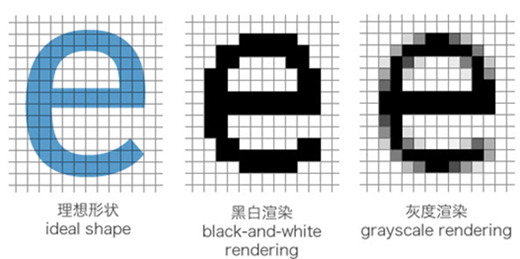##### 网站与APP开发中的字体设置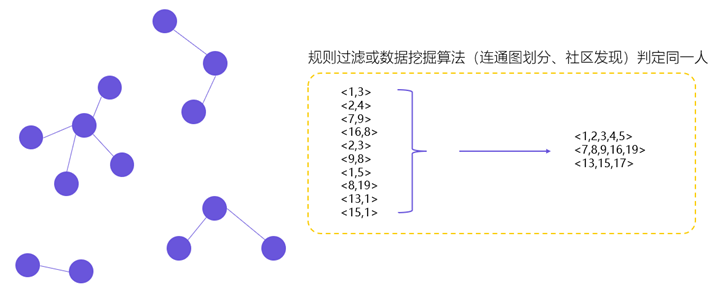##### 用户体系搭建之ID-Mapping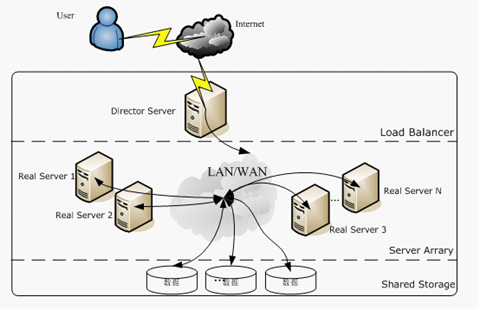## 2 Replies to “机器学习聚类算法之HDBSCAN”

1. 欧锦赛说道：

ax.set_xlim(120, 122)
ax.set_ylim(30, 33)

在我的电脑上跑最后的示例程序，得把上面两行代码注释掉才能真正出图，不然点会在图外，给其他小伙伴一个提醒。

2. gao说道：

您好请问这个程序运行的数据格式是怎么样的，我试了。txt,csv为什么都报错